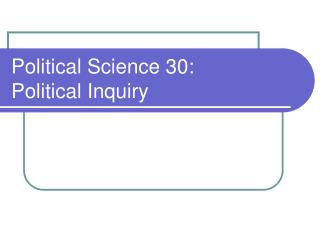Download PresentationPolitical Science 30: Political Inquiry

# Political Science 30: Political Inquiry

Download Presentation## Political Science 30: Political Inquiry

- - - - - - - - - - - - - - - - - - - - - - - - - - - E N D - - - - - - - - - - - - - - - - - - - - - - - - - - -
##### Presentation Transcript

1. Political Science 30:Political Inquiry

2. Linear Regression I:Scatterplots and Regression Lines • SAT scores and graduation rates • Looking at a scatterplot • Fitting a regression line • What does it mean? • Other factors that affect graduation rates • Confound: reputation • Other independent variables

3. SAT Scores and Graduation Rates • To test the hypothesis that colleges with smarter students have higher graduation rates, I will look at data from 148 colleges in the United States. SAT Scores Graduation Rates

4. SAT Scores and Graduation Rates

5. SAT Scores and Graduation Rates • We can summarize the direction and strength of a relationship between two variables by calculating “r,” the correlation. (Pollock, p. 171-174) • If we want to know more about the relationship, we can fit a “regression line” to the scatterplot.

6. SAT Scores and Graduation Rates

7. SAT Scores and Graduation Rates • A regression line summarizes how much the dependent variable (graduation rates) changes when the independent variable (SAT scores) increases. • The line gives you a “predicted value” of graduation rates for a college with a given average SAT score. • To make this prediction as good as possible, the line minimizes vertical distances between the line and the data points.

8. SAT Scores and Graduation Rates • Like all lines, a regression line can be summarized with this formula: y = a + b•x where: b = slope of line or “regression coefficient” a = the intercept, or the value of y when x=0

9. SAT Scores and Graduation Rates • The regression coefficient for the effect of SAT scores on graduation rates is 0.06. • This is the predicted effect on graduation rates when SAT scores go up by one unit. • This means that comparing one college to another college where average SATs were 100 points higher should lead to a graduation rate that is 6 percentage points higher.

10. Other Factors Affecting Grad Rates • The school’s reputation could be a confound: a school with a good rep might attract smart students and want to keep its reputation high by graduating them.

11. Other Factors Affecting Grad Rates

12. Other Factors Affecting Grad Rates Year of Founding SAT Scores Graduation Tuition Rates Student/Faculty Ratio